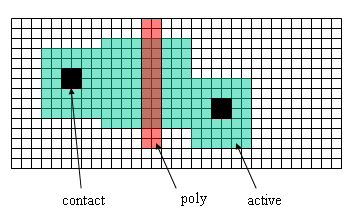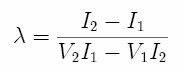# Microelectronics Assignment 4

Your report should be a Microsoft Word document or PDF. Supplemental computer files associated with the assignment (SPICE, spreadsheets, etc.) should be referenced in the main document and included in your submission, organized in some appropriate manner.

1. The diagram below shows a transistor (poly over n+) with a grid spacing of one λ square. Assume a process with λ = 0.3 µm. Find the transistor parameters (W, L, AD, PD, AS, PS, NRS, NRD). Assume the drain is the active area on the right.2. Show the netlist from the NMOS_IV transistor layout obtained in Assignment 3. Verify the values of AD, AS, PD, and PS using the dimensions of the source and drain. See example done in class.

3. Given the following information from an NMOS characteristic curve (VGS = 5 Volts):

 VDS (V) ID (µA) 2.1 237.85 4.5 326.76 5.0 328.76

You may find the following formula useful:1. Find the transconductance k and channel modulation λ for the simple (level 1) model that match the measured values in the saturation region of the table above. VT = 0.8 V.

2. Find the effective resistance of the transistor.

3. Calculate the current ID at VDS = 4 V for the level 1 model.

4. Work through tutorial 3 (see link below). Your report should include layout figures, schematic figures, and a report of Spice analysis.

Tutorial 3 – Design, layout, and simulation of a CMOS inverter – electric_tutorial_3_video.wmv (27:45)

5. Design an inverter with the NMOS transistor sized 6x2 (WxL), and the PMOS transistor sized so that the switch point is close to 2.5 V. Show a diagram of the resulting circuit and a copy of the netlist. This inverter should have ports and power node connections and an external symbol representation - like the example we showed in class.

Maintained by John Loomis, last updated 10 February 2014